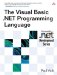# Methods

 <  Day Day Up  >

### Methods

A method is a set of executable statements. Methods come in two forms: functions, which return a value, and subroutines, which don't. The following example shows a function that adds two numbers and returns the result, and a subroutine that calls it and prints the result of the function.

` Module Test   Function Add(ByVal x As Integer, ByVal y As Integer) As Integer     Return x + y   End Function   Sub Main()     Console.WriteLine(Add(10, 20))   End Sub End Module `

#### Parameters

Methods can have parameters , which allow passing values to and from the method. Values passed to parameters are called arguments to the method. By default, arguments are passed to parameters by value, which means the argument is copied into the parameter when the method is entered. Arguments can also be passed to parameters by reference, which means that the parameter gets a pointer to the argument itself, rather than a copy. Any changes to a reference parameter are reflected in the original argument. In the following example, the Add subroutine does not return a value ”instead, it assigns the result value to the reference parameter Result . This changes the Result local variable in the Main subroutine.

` Module Test   Sub Add(ByVal x As Integer, ByVal y As Integer, _           ByRef Result As Integer)     Result = x + y   End Sub   Sub Main()     Dim Result As Integer     Add(10, 20, Result)     Console.WriteLine(Result)   End Sub End Module `

Parameters can be optional, with a default value that is supplied if no argument is given. Optional parameters must always be declared after required parameters. The following example declares the second parameter as optional, with a default value of 10. The Main subroutine prints the value 20.

` Module Test   Function Add(ByVal x As Integer, Optional ByVal y As Integer = 10) _       As Integer     Return x + y   End Function   Sub Main()     Console.WriteLine(Add(10))   End Sub End Module `

Parameters can also be parameter arrays . A parameter array is a parameter whose type is a special kind of array. A caller can pass zero or more arguments of the element type of the array, and they will be packaged into an array when the call is made. In the following example, the Add function now can add an arbitrarily large number of integers together.

` Module Test   Function Add(ByVal ParamArray Values() As Integer) As Integer     Dim Result As Integer = 0     For Index As Integer = 0 To Values.Length - 1       Result += Values(Index)     Next Index     Return Result   End Function   Sub Main()     Console.WriteLine(Add(10,20,30,40,50,60))   End Sub End Module `

Methods can be overloaded with different parameter lists. At compile time, the best overload for the given arguments will be chosen. If a "best" overload cannot be chosen , an ambiguity error will be given. Methods cannot be overloaded solely by return type. In the following example, the Add method is now overloaded with both an Integer and a Double version. The compiler will choose to call one or the other based on which one the arguments best match.

` Module Test   Function Add(ByVal x As Integer, ByVal y As Integer) As Integer     Return x + y   End Function   Function Add(ByVal x As Double, ByVal y As Double) As Double     Return x + y   End Function   Sub Main()     ` Calls Add(Integer, Integer)     Console.WriteLine(Add(10,20))     ` Calls Add(Double, Double)     Console.WriteLine(Add(12.3, 31.4))   End Sub End Module `

#### Declare Statements

It is sometimes necessary to call methods that exist outside the .NET Framework, such as Win32 APIs. These external methods are represented within the Framework by Declare statements. A Declare statement specifies the dynamic link library in which the external method resides and the method's parameters and return type. The following example shows a sample Declare statement that represents the Win32 API GetWindowsDirectoryA .

` Module Test   Declare Function GetWindowsDirectoryA Lib "kernel32" _     (ByVal Buffer As String, ByVal Size As Integer) As Integer   Sub Main()     Dim s As String     Dim Count As Integer     s = Space(256)    ` Fill the string with spaces     Count = GetWindowsDirectoryA(s, s.Length)     If Count < 256 Then       Console.WriteLine(s)     End If   End Sub End Module `

Declare statements can also provide an alias for the external method if the method name would conflict with an existing name or a different name is desired. The following example shows a sample Declare method for the GetWindowsDirectoryA external method that renames the method to GetWindowsDirectory .

` Module Test   Declare Function GetWindowsDirectory Lib "kernel32" _     Alias "GetWindowsDirectoryA" _     (ByVal Buffer As String, ByVal Size As Integer) As Integer   Sub Main()     Dim s As String     Dim Count As Integer     s = Space(256)    ` Fill the string with spaces     Count = GetWindowsDirectory(s, s.Length)     If Count < 256 Then       Console.WriteLine(s)     End If   End Sub End Module `
 <  Day Day Up  >The Visual Basic .NET Programming Language
ISBN: 0321169514
EAN: 2147483647
Year: 2004
Pages: 173
Authors: Paul Vick

Similar book on Amazon